All submissions of the EM system will be redirected to Online Manuscript Submission System. Authors are requested to submit articles directly to Online Manuscript Submission System of respective journal.

# Research

, Volume: 7( 3)

## Propellant-less Propulsion from Quantized Inertia.

*Correspondence:
ME McCulloch , Plymouth University, PL4 8AA, United Kingdom, Tel: +441752600600; E-Mail: mike.mcculloch@plymouth.ac.uk

Received: November 20, 2018; Accepted: December 13, 2018; Published: December 20, 2018

Citation: ME McCulloch. Propellant-less Propulsion from Quantised Inertia. J Space Explor. 2018;7(3):151

### Abstract

A new model for inertial mass, quantized inertia, that predicts galaxy rotation without dark matter, also predicts that a highly accelerated system surrounded by an asymmetric conductor will feel thrust in a new way, without having to store and expel propellant. This may already have been seen in the emdrive, which quantized inertia predicts quite well. Here it is shown that thrust effects from asymmetric capacitors (including the Woodward effect) can also be predicted to the right order of magnitude. The lack of a need to store heavy propellant implies much lighter launch systems and the possibility of interstellar travel.

### Keywords

Propellant-less propulsion; Quantized inertia; Inertial mass; Thrust; Dark matter

### Introduction

For many years there have been indications that thrust can be obtained from closed electrical systems. One example is the emdrive, a truncated metal cone in which microwaves are allowed to resonate. It has been shown  that such a system can show an unexplained thrust towards its narrow end, and this has been replicated by NASA . A similar system called a Mach Effect Thruster (MET) has been under development . Thrust can be obtained if an AC current is fed into a Piezoelectric Material (PZT) sandwiched between a thick conducting plate and a thin one. The thrust is always towards the thick plate. However, Woodward JF's  own hypothesis does not predict this thrust, being in error by several orders of magnitude .

A theory has been proposed called quantized inertia in which dynamics arise solely from a push on objects by the quantum vacuum, which can be made more intense by high acceleration (Unruh radiation) and made non-uniform in space either by matter (to explain gravity) or relativistic acceleration-dependent Rindler horizons (to explain inertia). The theory predicts galaxy rotation without dark matter and without any adjustment [5-8]. Quantized inertia implies that it is possible to produce new dynamics by artificially creating horizons which damp the quantum vacuum, making it inhomogeneous and so, able to push on objects. This kind of propulsion (a QI- or horizon-drive) would be propellant-less enabling the launch of much lighter spacecraft, and enabling interstellar travel since speeds close to c could be achieved without the need to also overcome the inertia of heavy fuel.

One well-known example of damping quantum fields is the Casimir effect  in which two parallel conducting plates are placed close together, and damp the quantum vacuum between them producing a net force inwards, pulling the two plates together. If we imagine a V-shaped structure instead of parallel plates then the quantum vacuum would be more damped at the narrow end of the V and the shape should move towards that end-down the gradient in the vacuum. If we now imagine energizing the vacuum, that should enhance this thrust. In quantized inertia, the way to energize the vacuum is to make particles or photons accelerate very fast, and so Unruh waves appear in their frame of reference.

The controversial emdrive is very much like this. It consists of a truncated conducting cone (a V shape) and microwaves are made to resonate within it at a very high resonant frequency . As expected from the analysis above it moves towards the narrow end where the Unruh-enhanced quantum vacuum should be damped the most. Indeed, quantized inertia predicts the thrust obtained from the emdrive quite well and predicts that an optical analog system might be more effective [10-12].

In this paper, it is shown that quantized inertia predicts the Woodward effect quite well, much better than the theory of Woodward, and it also predicts some anomalous thrusts obtained from asymmetric capacitors.

### Method

We start with Heisenberg's uncertainty principle which states that the uncertainty in momentum times the uncertainty in the position of a quantum particle must be greater than half the reduced Planck's constant .(1)

The momentum p can be defined as the time integral of the force F, so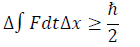(2)

We can now scale this up to consider, instead of one quantum ?, an energy-mass E that is composed of many quantum particles: the microwaves within the emdrive cavity or the electromagnetic fields in the Mach effect thruster. As a result, we now use the average force and uncertainty in position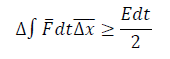(3)

Assuming that F does not vary with time, we get(4)

This is the force on the collection of mass-energy E due to quantum uncertainty, or jitter, and we assume that it is different in every direction. The unique point of view of quantized inertia is that the uncertainty in position (Δx) is affected by horizons which delete space beyond a certain distance, reducing Δx in that direction. This truncation can be due to either relativistic horizons due to acceleration, leading to inertia , or horizons made of matter which can lead to gravity . This truncation of space varies with direction and so Δx can vary with direction and therefore so can the force in Eq. 4 above. For the case of a system with a power input of P, the energy it contains at any one time E=Pτ where τ is the time the energy can linger before dissipating, which is equal to the number of 'bounces' of the energy back and forth in the system (Q) times the time taken for each bounce (length/c), so τ =QL/c , where L is the length of the system (because this is predictive for c being the speed of light, it implies that the bouncing phenomena is the electromagnetic field, not the matter). So(5)

For a particular system, the net force (thrust) is the difference in this force on opposite sides:(6)

### Results

1. For the emdrive

Equation 6 is identical to the formula derived, in a slightly different manner, but implying the same new physics (quantized inertia) for application to the emdrive in . FIG. 1 shows a schematic of the emdrive’s shape: a truncated cone with an input of resonating microwaves (represented by the white curve).

Quantized inertia (Eq. 6) predicts the observed emdrive thrusts quite well if the Δxs in the brackets is the distance to the walls of the emdrive cavity (half their diameter). For example, Shawyer's first emdrive  had a power input of 850 W, a Q factor of 5900, a length of 0.156 m and cavity end widths of 0.16 m and 0.1275 m. So the thrust prediction by Eq. 6 is(7)

The observed thrust was 16 mN, and, as predicted, it was towards the narrow end of the cavity. In a slightly, more complex form that takes account of the exact shape of the emdrive and the asymmetric distribution of dielectrics within it, quantized inertia is even more successfully predictive .

2. For the woodward drive

Here, Eq. 6 will be tested on a type of thruster that was first proposed and tested by Woodward JF  and later tested by Mahood TL, Fearn H and Tajmar M [4,13,14]. It is shown in a much-simplified schematic form by FIG. 2a, and an asymmetric capacitor is shown in FIG. 2b.

Figure 2: (a). The Woodward or Mach Effect Thruster as in (1st generation device) and its thrust towards the end with the thicker cap and (b) an asymmetric capacitor.

It consisted of a ring of six 0.01 μF, 3 kV capacitors on a PZT stack. Each capacitor was 0.8 cm square and 0.2 cm thick. The PZT stack was made of 40 PZT discs bonded together to make a cylindrical stack 2.5 cm tall (L) and 1.9 cm in diameter (the grey area). The black rectangles in the schematic represent two conductive (brass) end caps, the one on the left being much thicker. The weight of the device was monitored to determine the thrust (for more details see ). The power input P was 145 W and the frequency (f) of the AC input to the capacitors was 11 kHz. The prediction of  for the thrust using the Mach Effect Theory is(8)

which is 1000 times smaller than the thrust observed, which was of the order of 50 μN .

Now we can make a prediction using quantized inertia. It will be assumed that the thin end cap allows all Unruh waves through so in Eq. 6 Δxright=Θ (the only damping occurs at the cosmic scale, Θ) but the thicker end cap blocks all the Unruh waves so that damping occurs at a distance of Δxleft=L/2. This will produce an overestimate of the force since it is possible that not all the Unruh waves are blocked. As a result Eq. 6 simplifies to(9)

The Q (quality) factor was estimated at 60 because of a similar device that showed a dissipation rate of 2% and a statement that Q=60 for the thruster [14,15]. Using these values in Eq. 9 the thrust predicted by quantized inertia is(10)

This is close to the observed thrust which was on the order of 50 μN . There are also uncertainties in the assumed value for Q and in the observed thrust, which was given as an order of magnitude suggesting it could be a factor of 10 different. As mentioned above it may be that it is the em signal, not the vibrating solid matter, that is crucial, but the Q value is similar. According to , the quality factor of a cavity is Q=2πfE/P where f is the resonant frequency of the cavity.

In this case, f=c/L=3 × 108/0.025=1.2 × 1010 Hz where L is the plate-plate distance and c is the speed of light. E is the energy stored in the capacitor, given by E=0.5CV2 where capacitance C=εA/d=8.85 × 10-12 × 0.0082/0.025=2.3 × 10-14 F and V=3000 V. In this case then Q=2π × 1.2 × 1010 × 0.5× 2.3 × 10-14 × 30002/145=53.8. So this electromagnetic Q is within the range of those already discussed.

TABLE 1 shows the results for other tests of the 'sandwich-type' Woodward device that has so far been comprehensively described in the literature (most of the published papers do not contain enough information about P and Q). Column 1 lists the source paper, column 2 shows the power input and column 3 shows the quality factor (a range of values). Column 4 shows the AC frequency input. Column 5 shows the observed thrust. Column 6 shows the thrust predicted by Woodward's theory. Column 7 shows the thrust predicted by quantized inertia (Eq. 9), a range of values because of the uncertainty in Q.

Paper Power Q f Fobs FWoodward FQI
(W)   kHz ( µN ) ( µN ) ( µN )
Mahood, 1999 145 602-1903 11 50 0.05 29-92
Fearn et al., 2013 63 4 60-190 32.35 1.5 0.1 12.6-40

Table 1: Results for the 'sandwich-type' woodward device.

The superscripts: 1=First generation device, 2=Q estimated , 3=Q estimated , 4=Assuming the power input was as for .

It is clear from the Table that Woodward's theory underestimates the thrust by between a factor of 10 and 1000. Quantized inertia predicts the thrusts better. It agrees with the result in Mahood , within its uncertainties. It overestimates the second thrust value, but this might be expected since this analysis assumed that the thick cap completely blocks Unruh waves and the thin cap does not block them at all, so the predicted gradient in the Unruh radiation and the resulting thrusts are likely to be over-estimates.

3. For asymmetric capacitors in vacuum

In 2004 NASA (, as reported in ) tested an asymmetric capacitor in a high vacuum of 5.5 × 10-5 Torr. The setup was similar in some ways to the Woodward device, being an asymmetric capacitor, but with discharge sparks replacing the AC signal. The separation of the plates was 0.0635 m and the plates were 0.03 m wide. The applied voltage was 44kV and the current was less than 5 mA (this value had to be inferred by Martins AA, et al ). Upon a discharge, the system moved in an unspecified direction with a force of 14 mN. This is similar to the emdrive or the Woodward effect above since it is a large pulse of energy in an asymmetric conducting structure, but instead of exciting recoil mass with an AC signal, there is a DC signal applied. Again we can apply quantised inertia using Eq. 9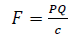(11)

According to McCulloch ME  the quality factor of a cavity is Q=2πfE/P where f is the resonant frequency of the cavity, in this case, f=c/L where L is the plate-plate distance and c is the speed of light. E is the energy stored In the capacitor, given by E= 0.5CV2, so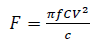(12)

The capacitance C of two parallel plates is given by C=εA/L where ε is the electric constant (ε=8.85 × 10-12 m-3 kg-1 s4 A2), A is the plate area and L is the plate-plate distance (0.0635 m) and f=c/L. So we have(13)

So that the predicted force is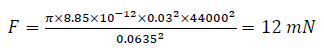(14)

This is close to the observed thrust, which was 14 mN. Although quantized inertia predicts that the direction of thrust should be towards the larger capacitor plate, the observed direction was not explicitly reported .

### Discussion

One way to vary or enhance the thrust obtained, and thereby provide a test of this particular explanation, would be to vary the frequency of the applied signal around the resonant frequency of the cavity. The maximum thrust should occur when the Unruh waves seen in the PZT are just the right wavelength so that the thick brass cap is at an anti-node and Unruh-damping is therefore maximized. The wavelength of the Unruh radiation is given by λ=8c2/a, (see for example  ). It may also be possible in this way to reverse the thrust by varying the frequency so that the thick brass cap or plate is at a node and the thin brass cap or plate is at an anti-node. Note  used a symmetrical arrangement of the brass masses and, as QI would predict, there was no thrust.

### Conclusion

It has been shown that when electrical energy is focused into a small volume in an asymmetric conductive structure, such as an emdrive, Mach effect thruster or asymmetric capacitor, then an unexpected thrust is seen towards the side with more ability to damp em (Unruh) waves.

It is shown here that examples of all three types of thrusters can be predicted quite well by a theory called quantized inertia, as being due to asymmetrical damping of the quantum vacuum (specifically Unruh radiation) leading to a gradient in the Unruh field that pushes on the object. The predictions are of the correct order of magnitude in all three cases. This then suggests the possibility of propellant-less propulsion, enabling easier launch and interstellar travel.

### References

###### Citations : 241

Journal of Space Exploration received 241 citations as per Google Scholar report

#### Indexed In

• Index Copernicus
• Open J Gate
• China National Knowledge Infrastructure (CNKI)
• Cosmos IF
• Directory of Research Journal Indexing (DRJI)
• Secret Search Engine Labs

View More

#### For Librarians